# Switch Open Data .ch and .li Domains

Hi All,

Switch the Swiss Registrar for .ch and .li Domains has published the Domains on a Open Data Policy.
You can download the top 1'000 Domains or use a Zone Transfer

Switch Open Data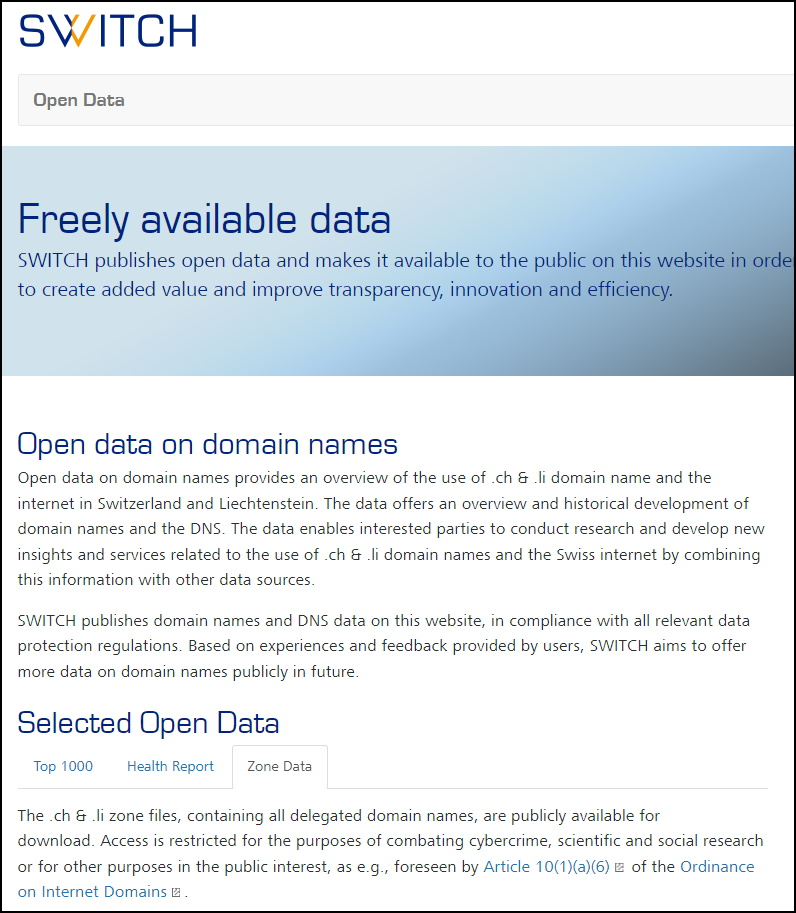You need to create the TSIG Key # filename ch_zonedata.keykey tsig-zonedata-ch-public-21-01 { algorithm hmac-sha512; secret "stZwEGApYumtXkh73qMLPqfbIDozWKZLkqRvcjKSpRnsor6A6MxixRL6C2HeSVBQNfMW4wer+qjS0ZSfiWiJ3Q=="; }; then use dig to initiate the Zone Transfer dig -k ch_zonedata.key @zonedata.switch.ch +noall +answer +noidnout +onesoa AXFR ch. > ch.txt Looks like this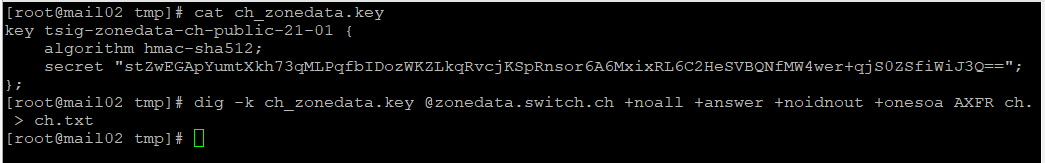I have used psftp.exe to transfer the ch.txt File to my Computer

open 172.21.175.80
cd /var/tmp
lcd E:\
get ch.txt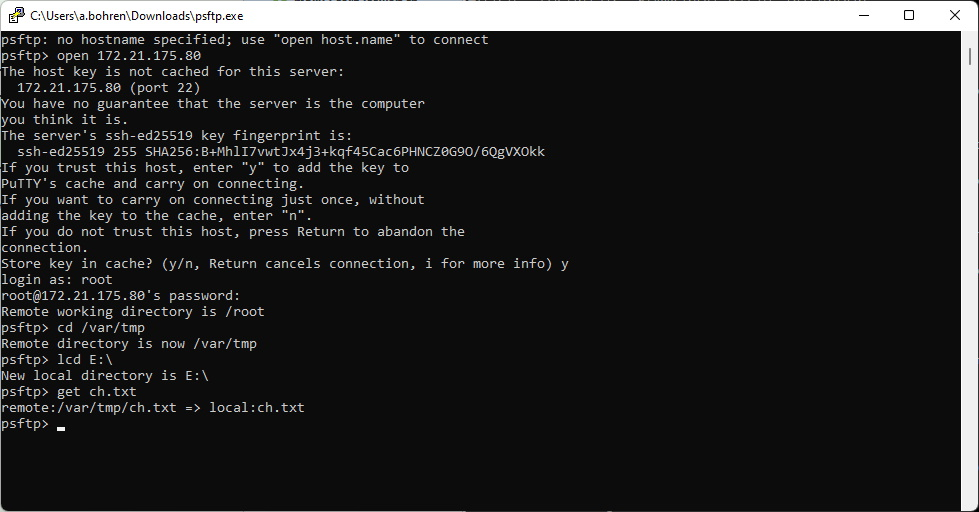The Zone File looks like "TAB" separated. But is not consistent in any way.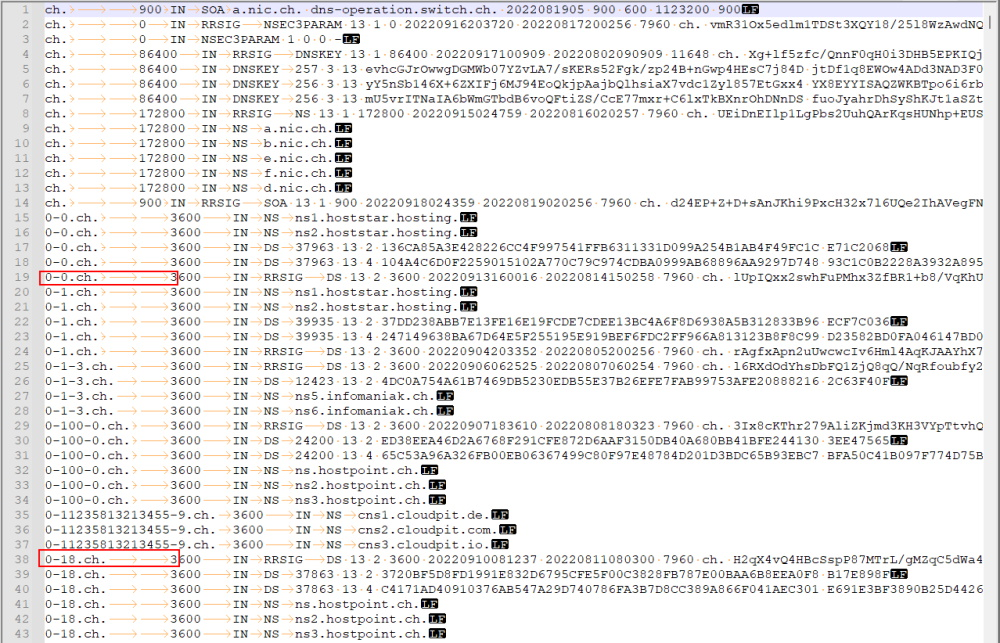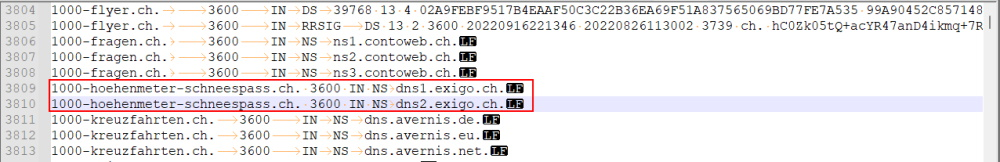It took me quite a while to write a performant PowerShell Script that fixes all these "issues" in the .ch Zone File. ############################################################################### # Fix Tab in .ch Zone File # 30.08.2022 Andres Bohren / https://blog.icewolf.ch ###############################################################################$INfile = "E:\ch.txt"
$OUTfile = "E:\ch_psedit.txt"$reader = new-object system.IO.StreamReader($INfile)$writer = new-object system.IO.StreamWriter($OUTfile, 0)$Start = Get-Date
$ArrayDomains = New-Object System.Collections.ArrayList #$ArrayDomains = @()
$Int = 0 while ($line = $reader.ReadLine()) { # do your thing$Int = $Int + 1$Line = $Line.Replace("ttt","t")$Line = $Line.Replace("tt","t")$Line = $Line.Replace(".ch. 3600",".ch.t3600")$Line = $Line.Replace(".ch. 3600",".ch.t3600")$Line = $Line.Replace("3600 IN RRSIG","3600tINtRRSIG")$Line = $Line.Replace("3600 IN DS","3600tINtDS")$Line = $Line.Replace("3600 IN NS","3600tINtNS")$Line = $Line.Replace("3600 IN","3600tIN")$Line = $Line.Replace("INtDS ","INtDSt")$Line = $Line.Replace("INtNS ","INtNSt")$Line = $Line.Replace("IN RRSIG","INtRRSIG")$Line = $Line.Replace("IN DS","INtDS")$Line = $Line.Replace("IN NS","INtNS")$Line = $Line.Replace("INtDS ","INtDSt")$Line = $Line.Replace(".ch. 900 IN NSEC3",".ch.t900tINtNSEC3")$Line = $Line.Replace(".ch. 900 IN RRSIG",".ch.t900tINtRRSIG")$Line = $Line.Replace("INtNSEC3 ","INtNSEC3t")$Line = $Line.Replace("INtRRSIG ","INtRRSIGt")$Line = $Line.Replace(".ch. 900 IN",".ch.t900tIN")$Line = $Line.Replace("INtRRSIG DS","INtRRSIGtDS")$Writer.WriteLine("$Line")$Domain = $Line.Split("t")$Domain = $Domain.Substring(0,$Domain.Length -1)
If ($Int%10000 -eq 0) { Write-Host "Domain:$Domain [$int]" } }$End = Get-Date
$Timespan = New-TimeSpan -Start$Start -End $End$Timespan
Write-Host "Lines processed: $INT" -ForegroundColor Green$reader.close()
$writer.Close()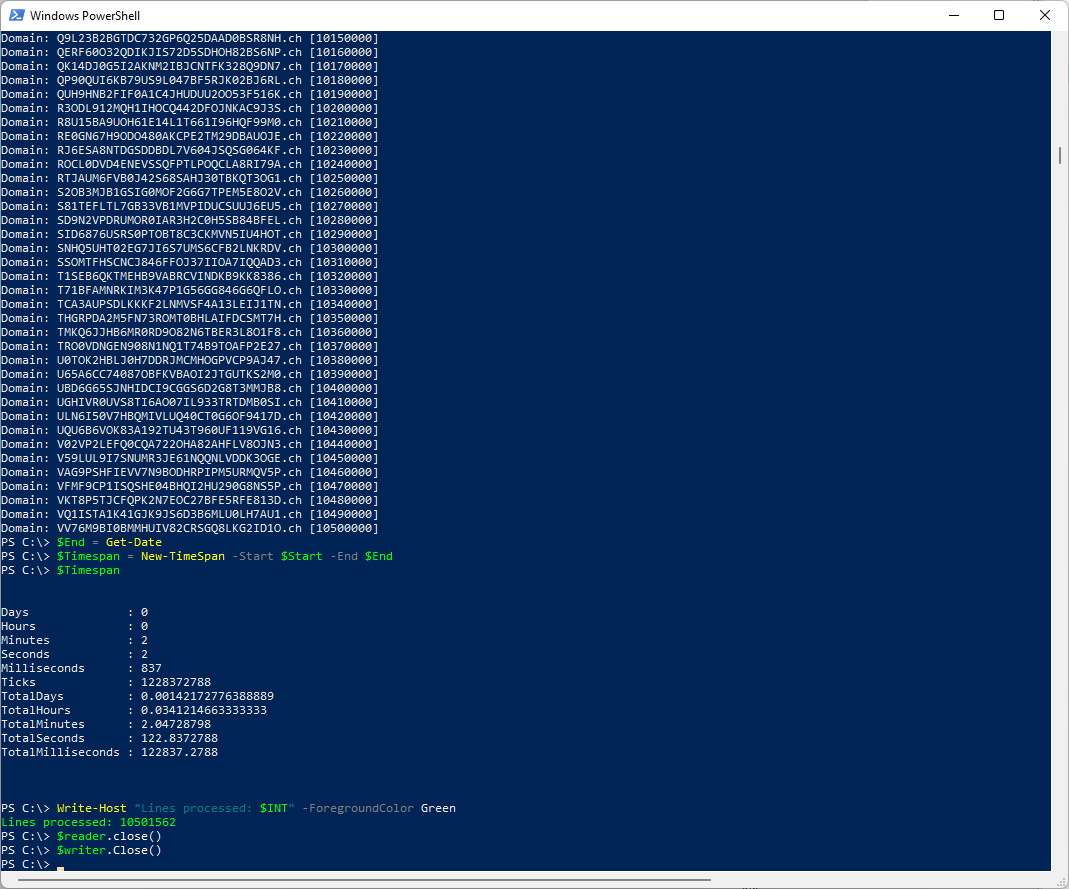This Script converts the File in TAB Separated Columns and adds CRLF at the end of the Line.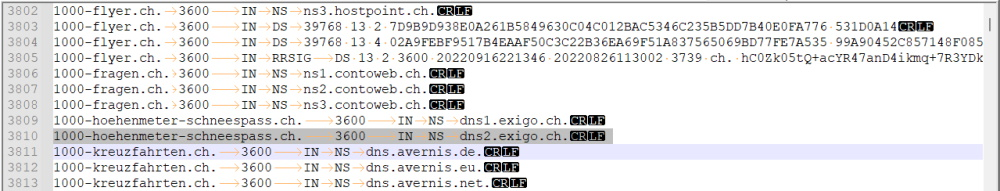The File still has over 10'000'000 Lines. As you can see there are multiple Lines for the same Domain. We need to find a way to make them Unique. I use the "SQL Server Import and Export Wizard" to import the File into a SQL Database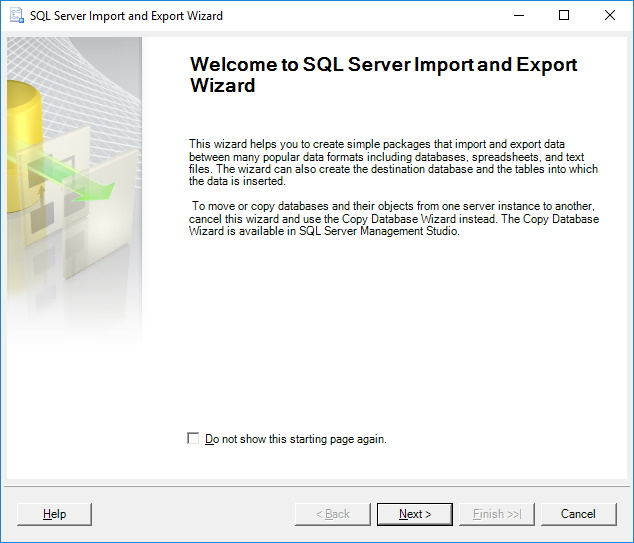Source: Flat File Source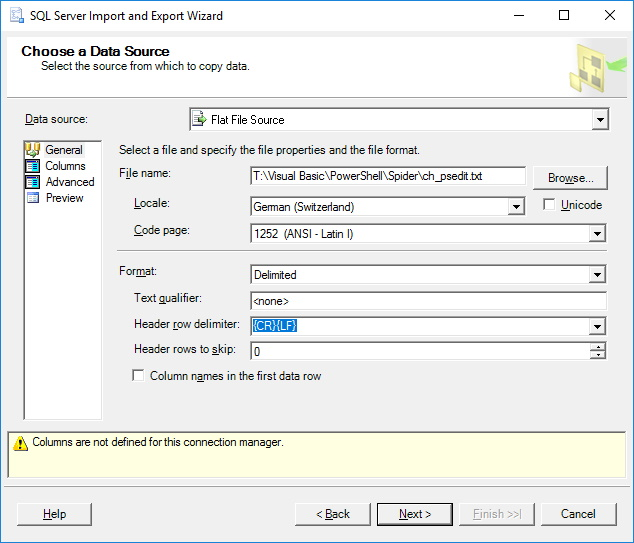Set the Names of the Columns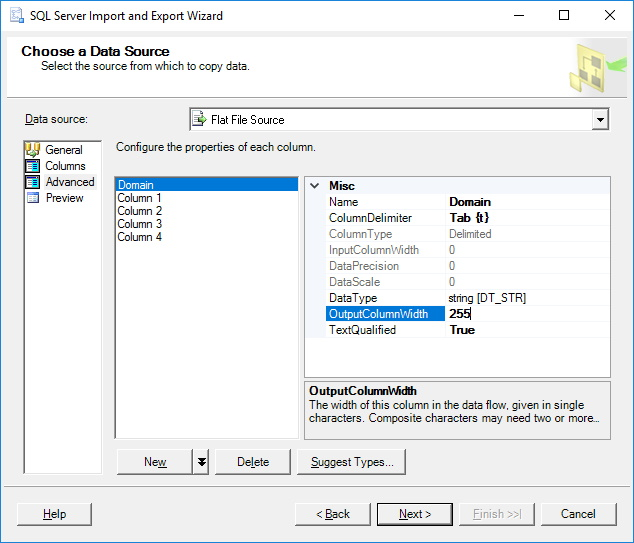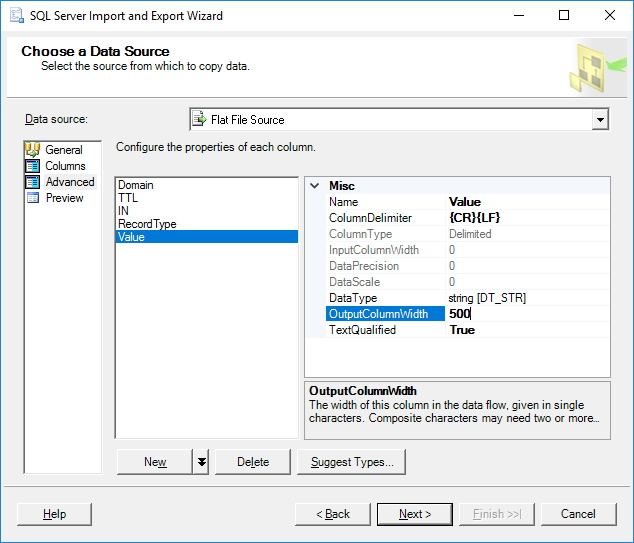Preview looks fine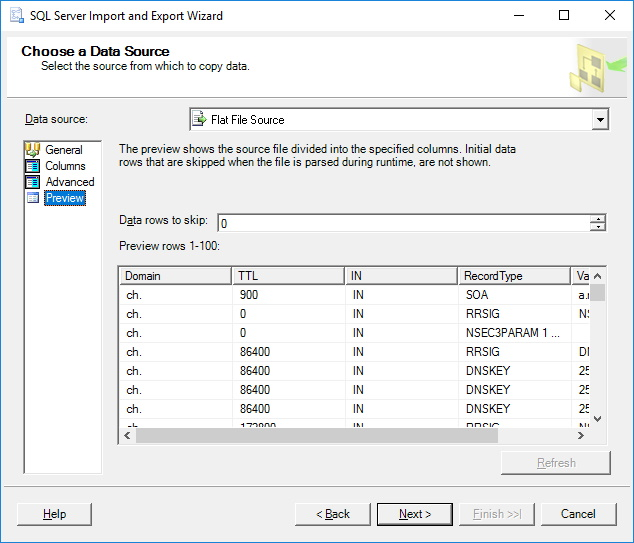Destination: SQL Server Express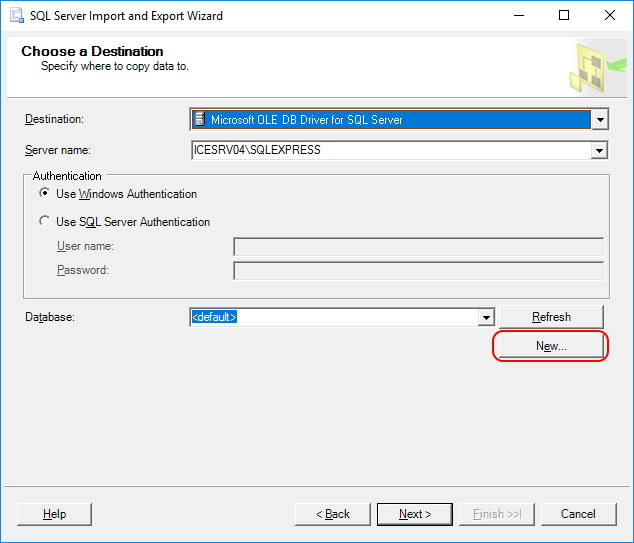Create new Database: db_domain_raw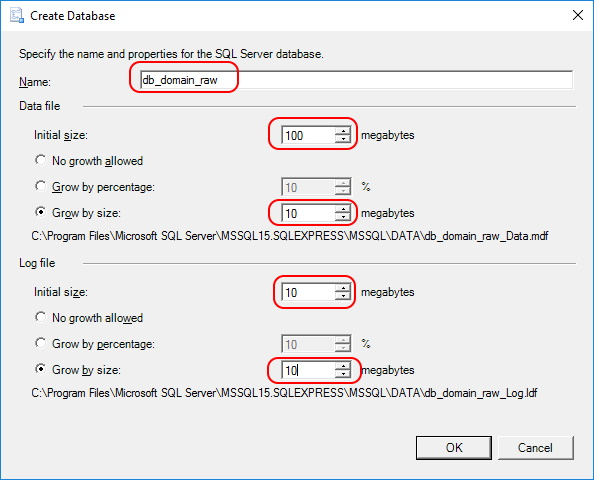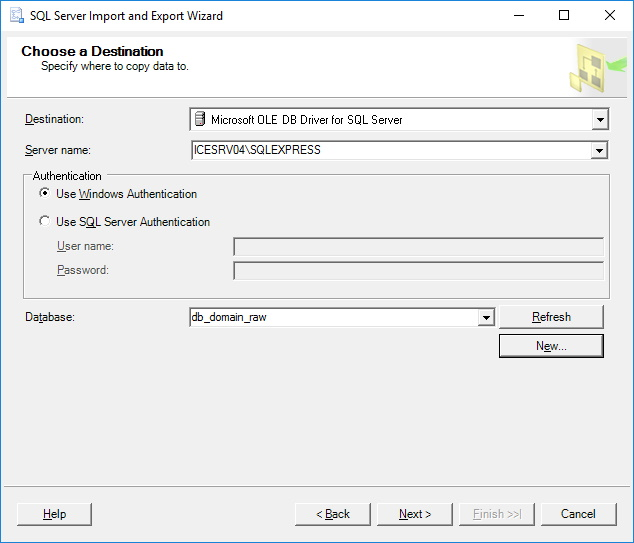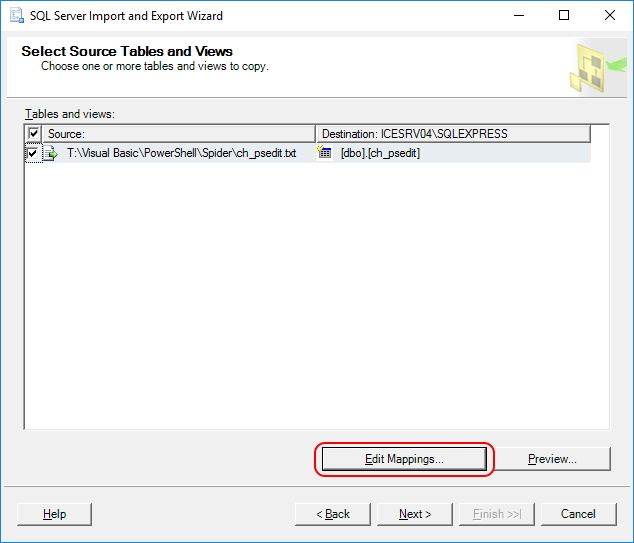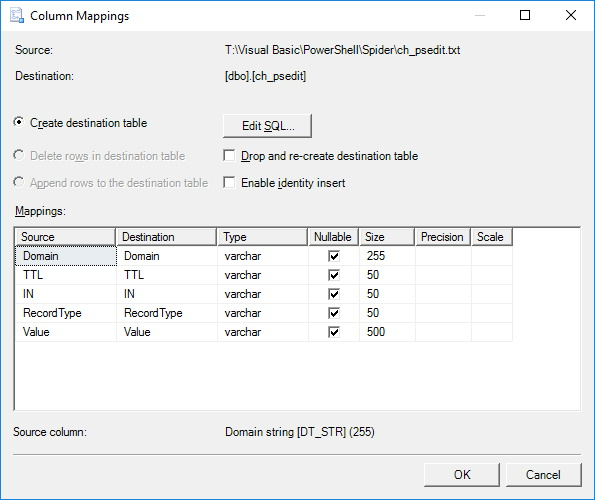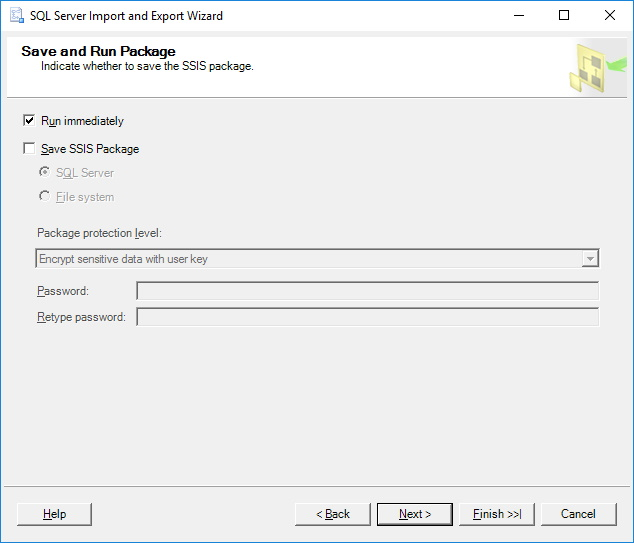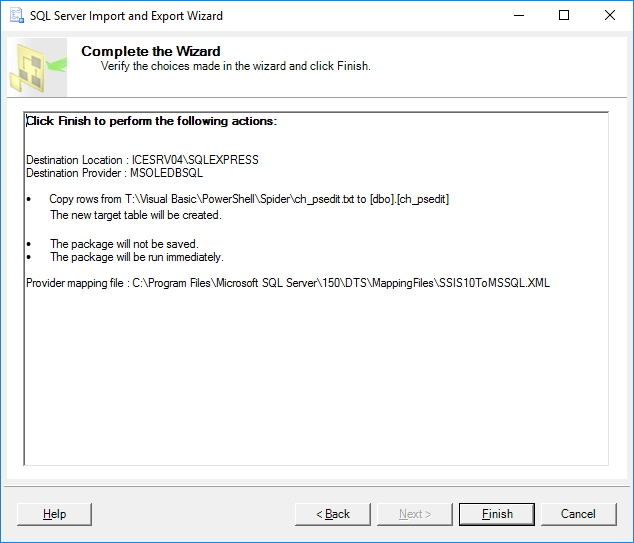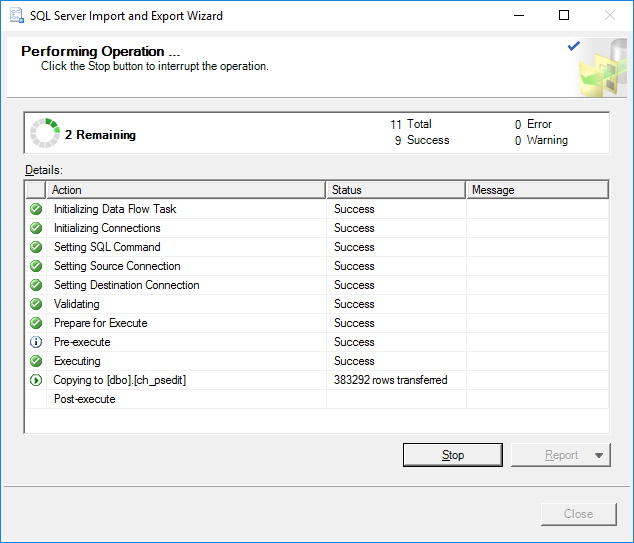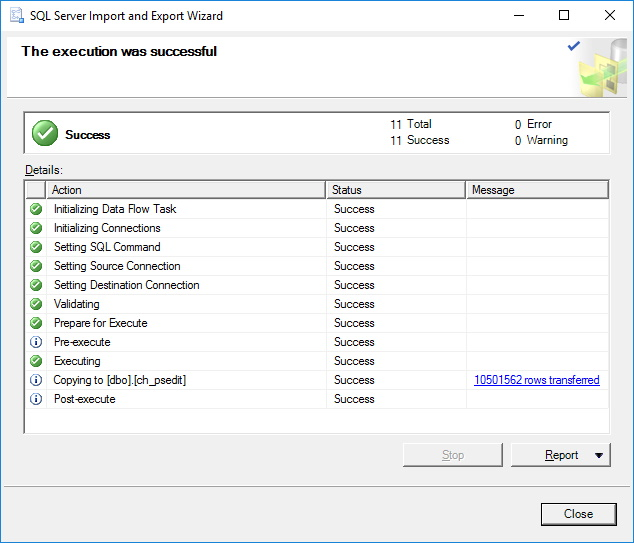Now i can use the "Select Distinct" to find out Unique Domains USE db_domain_raw GO SELECT DISTINCT RTRIM(Domain) as Domain FROM ch_psedit WHERE RecordType = 'NS' ORDER BY Domain ASC After two Minutes it's clear that there are about 2.4 Mio .ch Domains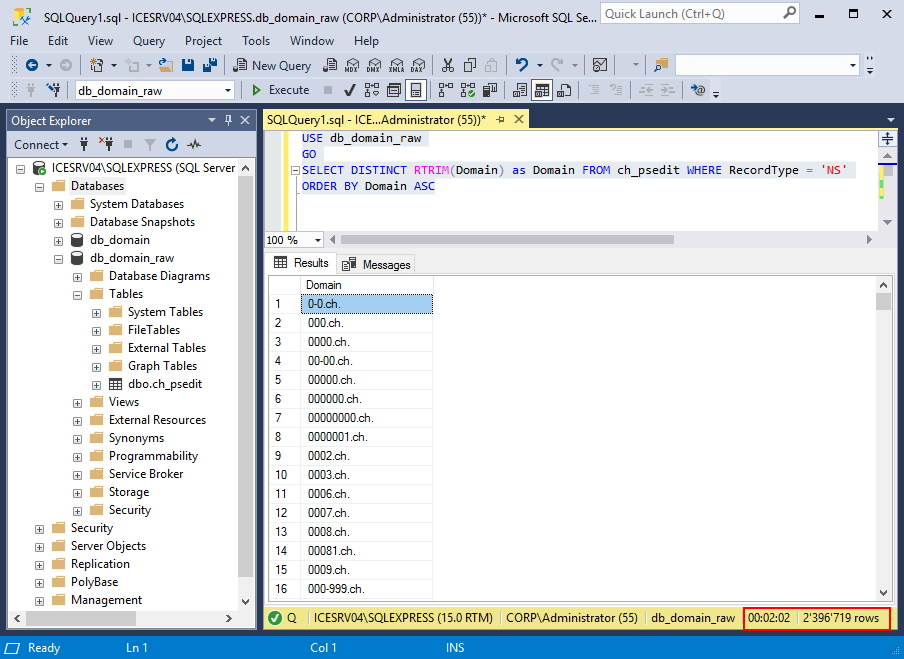Regards
Andres Bohren